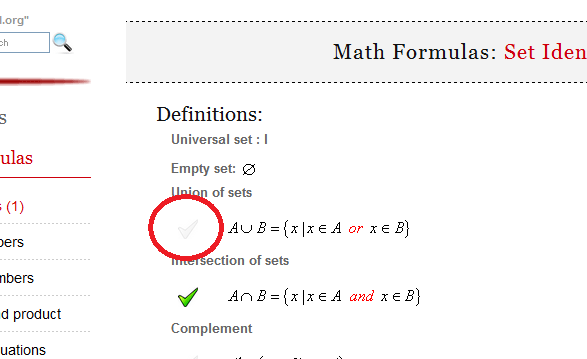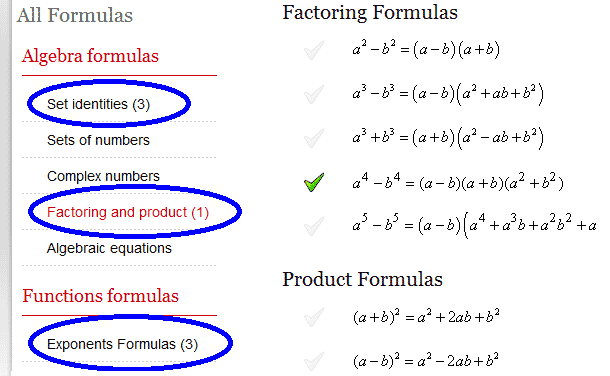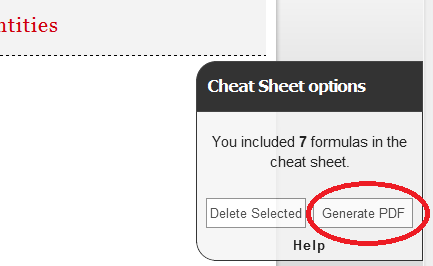Math Calculators, Lessons and Formulas

It is time to solve your math problem

mathportal.org

You included 0 formulas in your custom cheat sheet.

Cheat Sheet options

## How to generate cheat sheet ?

To create cheat sheet first you need to select formulas which you want to include in it. To select formula click at picture next to formula.You can choose formulas from different pages.After you have selected all the formulas which you would like to include in cheat sheet, click the "Generate PDF" button.# Math Formulas: Lines in two dimensions

### Line forms

Slope y-intercept form:

 $$y = mx+b$$

Two point form:

 $$y - y_1 =\frac{y_2-y_1}{x_2 - x_1} (x - x_1)$$

Point slope form:

 $$y - y_1 = m(x - x_1)$$

Intercept form

 $$\frac{x}{a} + \frac{y}{b} = 1~,~(a,b \ne 0)$$

Normal form:

 $$x\cdot \cos\Theta + y\cdot \sin\Theta = p$$

Parametric form:

 \begin{aligned} x &= x_1 + t\cdot \cos\alpha \\ y &= y_1 + t\cdot \sin\alpha \\ \end{aligned}

Point direction form:

 $$\frac{x - x_1}{A} = \frac{y - y_1}{B}$$

where $(A,B)$ is the direction of the line and $P_1(x_1, y_1)$ lies on the line.

General form:

 $$Ax + By + C = 0~,~(A\ne 0 ~\text{or}~B \ne 0)$$

### Distance

The distance from $A\,x + B\,y + C = 0$ to $P_1(x_1, y_1)$ is

 $$d = \frac{|A\,x_1 + B\,y_1 + C|}{\sqrt{A^2 + B^2}}$$

### Concurrent lines

Three lines

 \begin{aligned} A_1x + B_1y + C_1 &= 0 \\ A_2x + B_2y + C_2 &= 0 \\ A_3x + B_3y + C_3 &= 0 \end{aligned}

are concurrent if and only if:

 $$\begin{vmatrix} A_1 & B_1 & C_1 \\ A_2 & B_2 & C_2 \\ A_3 & B_3 & C_3 \\ \end{vmatrix} = 0$$

### Line segment

A line segment $P_1P_2$ can be represented in parametric form by

 \begin{aligned} x &= x_1 + (x_2 - x_1)t \\ y &= y_1 + (y_2 - y_1)t \\ & 0 \leq t \leq 1 \end{aligned}

Two line segments $P_1P_2$ and $P_3P_4$ intersect if any only if the numbers

 $$s = \frac{ \begin{vmatrix} x_2 - x_1 & y_2 - y_1 \\ x_3 - x_1 & y_3 - y_1 \end{vmatrix}} { \begin{vmatrix} x_2 - x_1 & y_2 - y_1 \\ x_3 - x_4 & y_3 - y_4 \end{vmatrix}} ~~ \text{and} ~~ t = \frac{ \begin{vmatrix} x_3 - x_1 & y_3 - y_1 \\ x_3 - x_4 & y_3 - y_4 \end{vmatrix}} { \begin{vmatrix} x_2 - x_1 & y_2 - y_1 \\ x_3 - x_4 & y_3 - y_4 \end{vmatrix}}$$

satisfy $0 \leq s \leq 1$ and $0 \leq t \leq 1$.